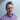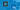Carl Rippon

# Using Currying to Pass Additional Data to React Event Handlers

June 02, 2020
typescriptreact

## An example

There are cases when we want to pass additional data to a React event handler. Consider the example below:

``````const List = ({
items,
}: {
items: string[],
}) => {
const handleClick = () => {
console.log(
"How do I get the item related to the button?"
);
};
return (
<ul>
{items.map((item) => (
<li key={item}>
<span>{item}</span>
<button onClick={handleClick}>
Log
</button>
</li>
))}
</ul>
);
};``````

We want to pass the list item data to `handleClick`.

We could use an inline anonymous function handler that calls `handleClick` passing in the list item:

``````const List = ({
items,
}: {
items: string[],
}) => {
const handleClick = (item: string) => {    console.log(item);
};
return (
<ul>
{items.map((item) => (
<li key={item}>
<span>{item}</span>
<button
onClick={() => handleClick(item)}          >
Log
</button>
</li>
))}
</ul>
);
};``````

There is an arguably nicer approach, though, called currying. First, let’s understand what currying is.

## What is currying?

Currying is an approach when functions receive one argument at a time.

``const func = (a, b) => { ... }``

A currying approach would be:

``const func = (a) => (b) => { ... }``

## An improved `handleClick`

We can use a currying `handleClick` as follows:

``````const List = ({
items,
}: {
items: string[],
}) => {
const handleClick = (item: string) => () => {    console.log(item);
};
return (
<ul>
{items.map((item) => (
<li key={item}>
<span>{item}</span>
<button onClick={handleClick(item)}>            Log
</button>
</li>
))}
</ul>
);
};``````

So, the click handler is the second function. The first function passes the list item to the handler.

Neat!

## Another example

Another example where currying is useful is form field change handlers. Typically we may have something like this where each field has a separate change handler:

``````<form onSubmit={handleSubmit}>
<input
type="text"
value={values.name}
onChange={handleNameChange}  />
<input
type="text"
value={values.email}
onChange={handleEmailChange}  />
<textarea
placeholder="Enter some notes"
value={values.notes}
onChange={handleNotesChange}  />
<button type="submit">Save</button>
</form>``````

Currying can help us condense the change handlers into a single handler:

``````const handleChange = (fieldName: string) => (  e: React.ChangeEvent<    HTMLInputElement | HTMLTextAreaElement  >) => {  setValues({
...values,
[fieldName]: e.currentTarget.value,
});
};

return (
<form onSubmit={handleSubmit}>
<input
type="text"
value={values.name}
onChange={handleChange("name")}    />
<input
type="text"
value={values.email}
onChange={handleChange("email")}    />
<textarea
placeholder="Enter some notes"
value={values.notes}
onChange={handleChange("notes")}    />
<button type="submit">Save</button>
</form>
);``````

The change handler is the second function. The first function passes the field name to the handler.

Neat!

These examples can be found in CodeSandbox at https://codesandbox.io/s/curry-event-handlers-q38xq?file=/src/index.tsx